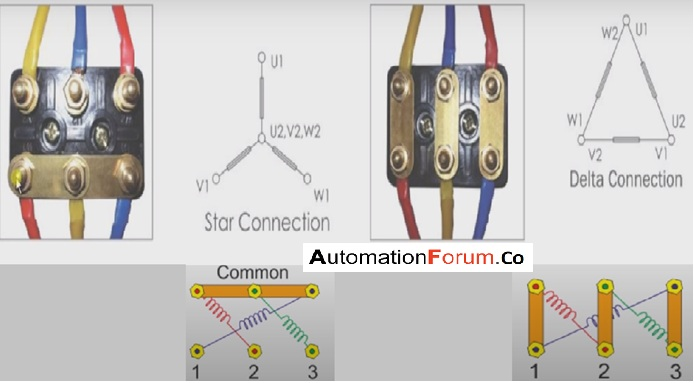# How to connect a three-phase motor in star and delta connectionIn a three-phase, motor the delta and star connection is the way in which the coils are connected. Star and delta connection is used to reduce the complexity of the wires that are required for a three-phase system connection. In the case of a normal three-phase connection, we would need more wire and that’s why we use this type of connection

## Star connection in a motor

A three-phase motor will have three coils and it can be connected to the supply and the coil would have connections in both sides, in the below image we can see the R, Y, and B, it is the coils and they are connected to the supply and we can also see the three terminals that are interconnected or shorted in the image. The below image shows the star connection of a motor in this the upper end of the coil is connected to the supply so the R, Y, B is connected the phase line and the end part of the coil is connected to each other and because of this connection, the voltage will be zero in this part. In a star connection, the same ends of the three coils are connected to create a neutral point.

Star connection is used only when we require less power and it would only give low power when compared to the delta connection because the three terminals are shorted and it would become neutral. So there will be three winding in this connection and each winding would get 220 supply. So because of this, we use star connection for low loads. Star connection is mostly used in places where we require a neutral point and two separate voltages.

### Delta connection of the motor

Delta connection is done for high loads, in the delta connection the terminals are connected in a different way than the star connection and it is shown in the below image and we can see that the opposite ends of three coils are connected together. This type of terminal connection would give 440v to the windings because for each winding there is two-phase and because of that each winding has 440v and due to this there will an increase in torque and that’s why delta connection is used for heavy loads. A delta connection is done where the neutral is not needed and also in places that require the control of third harmonics.

Scroll to Top